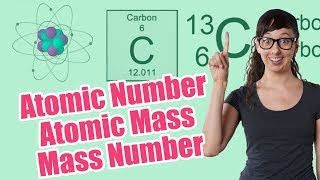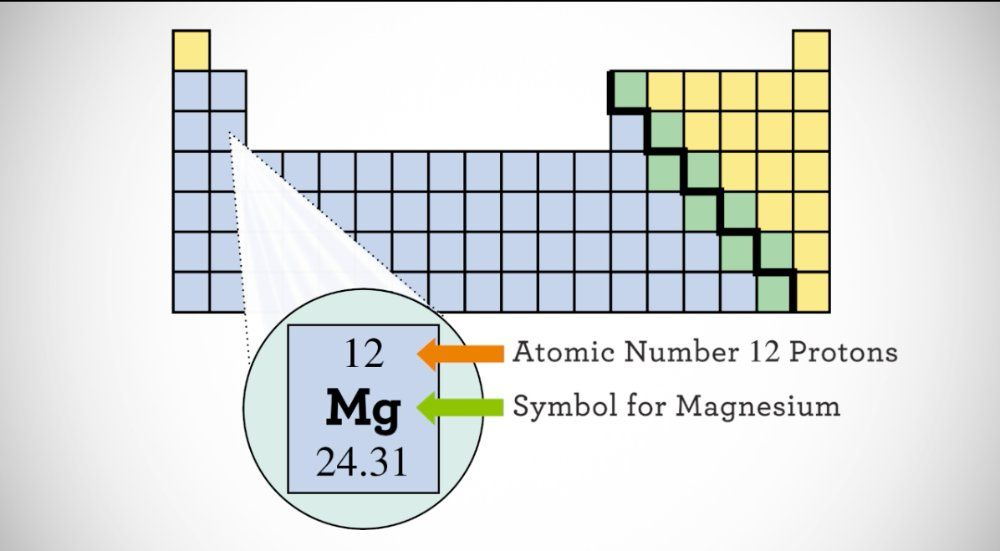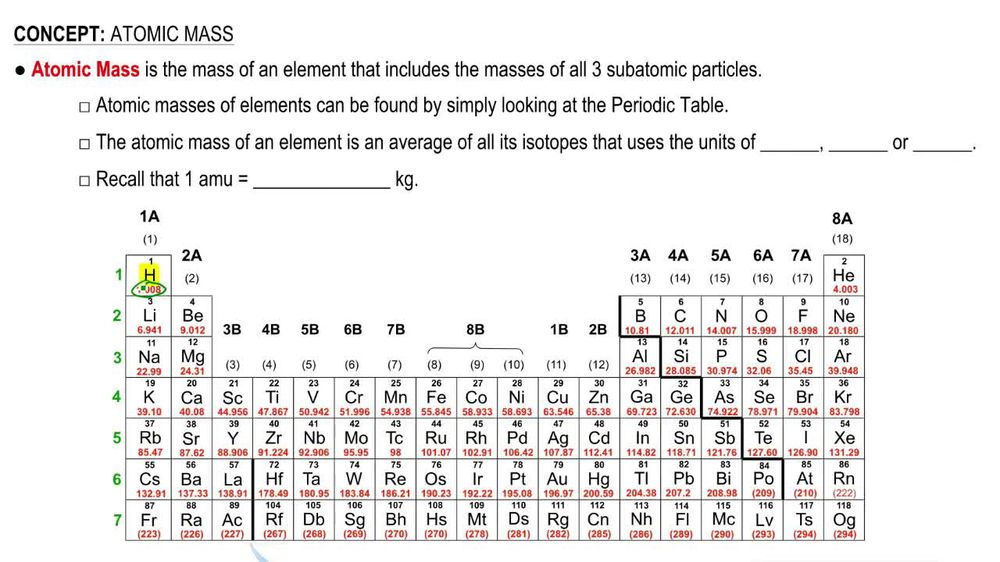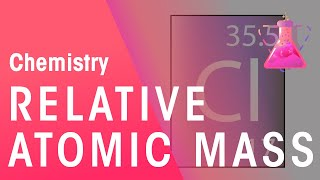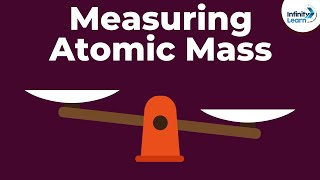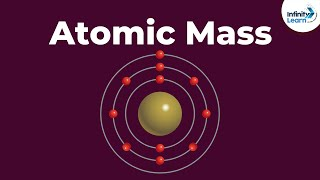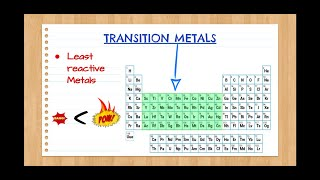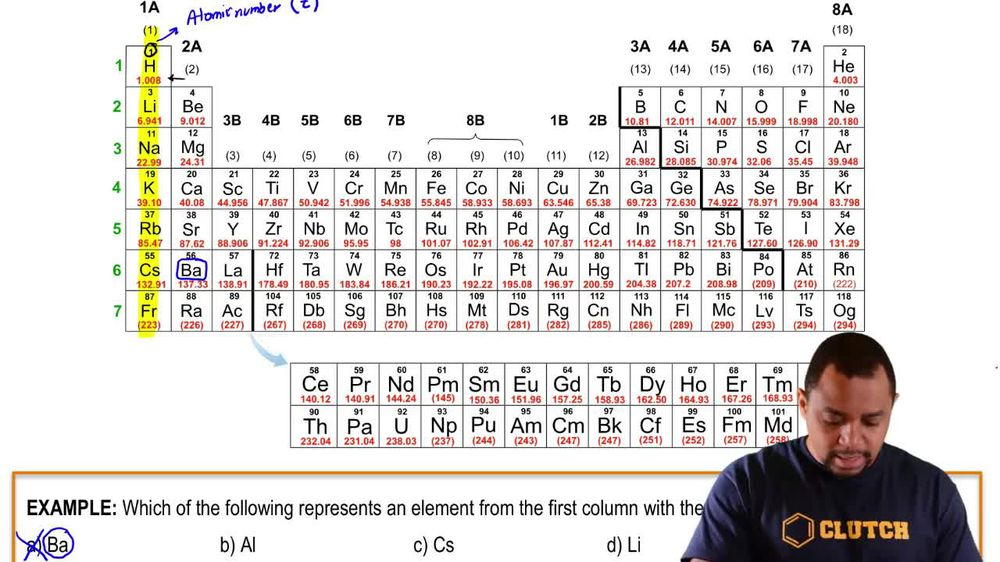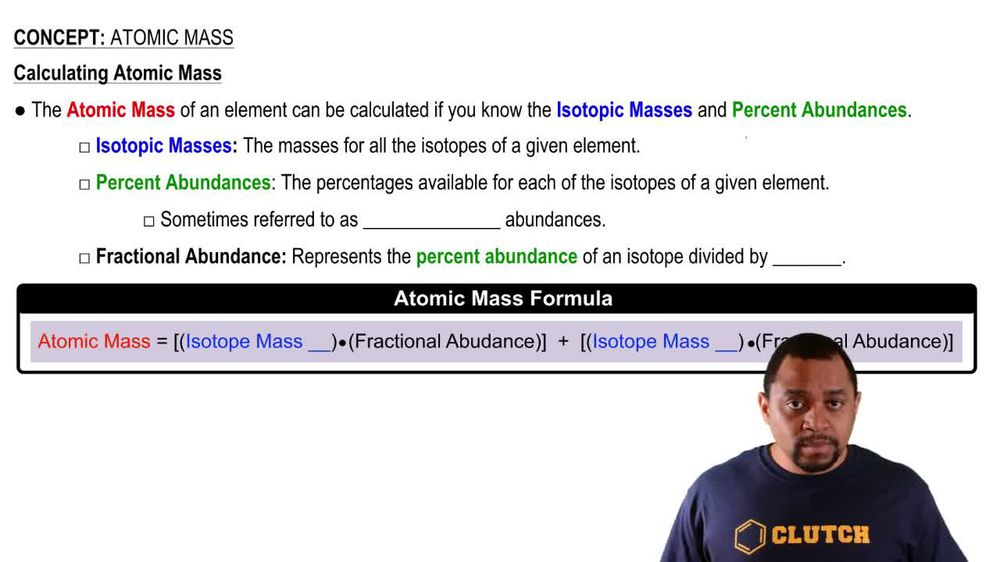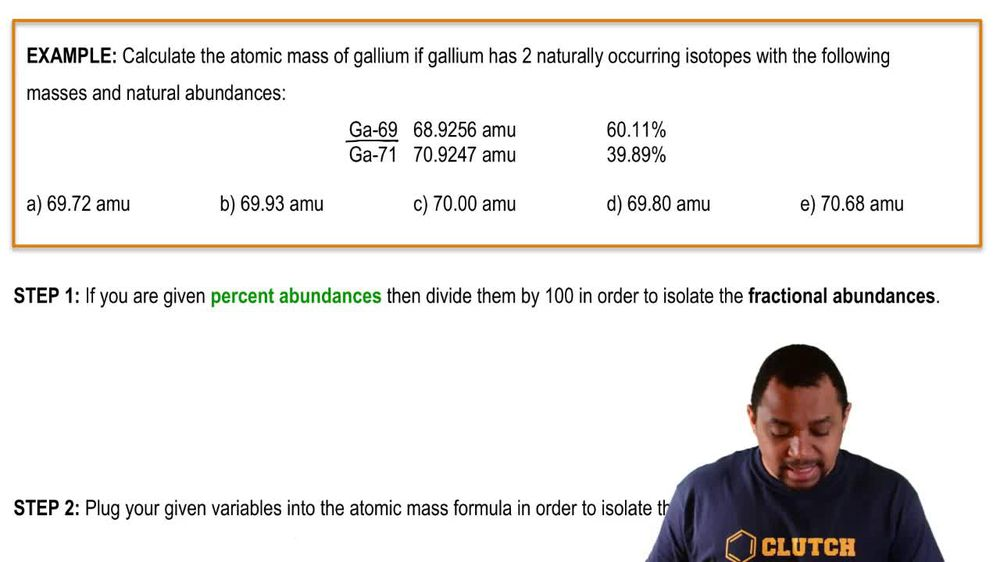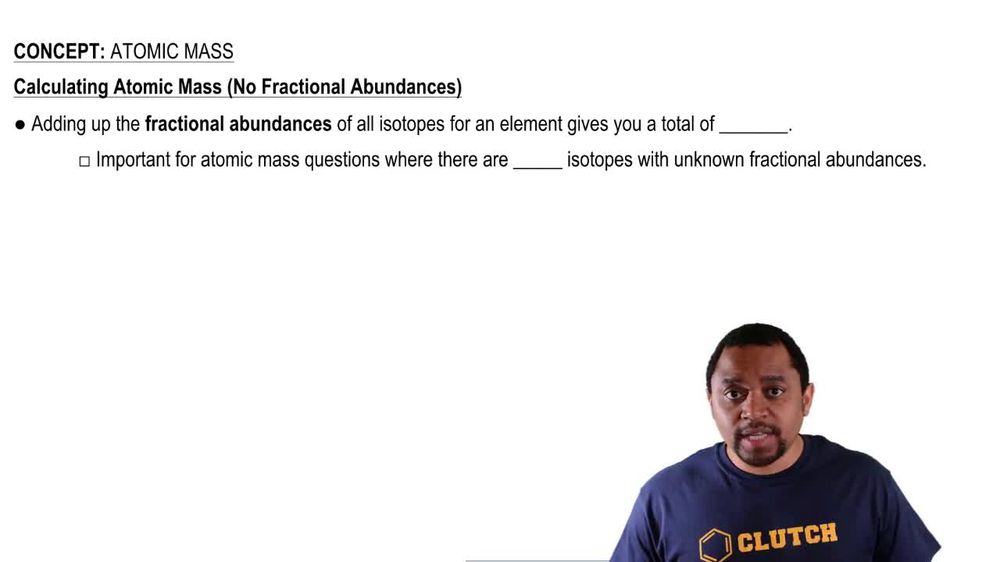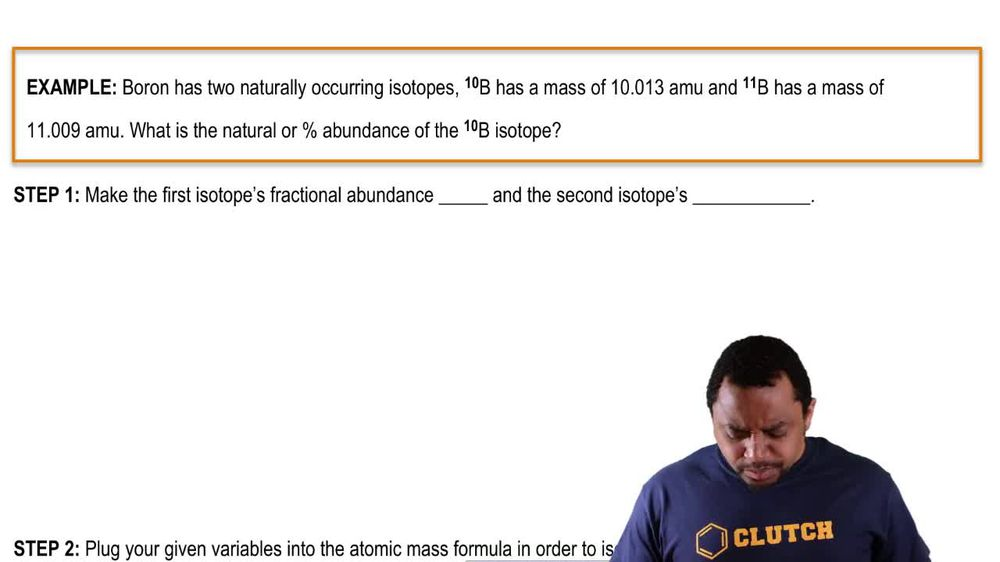Start typing, then use the up and down arrows to select an option from the list.
1. 2. Atoms & Elements2. Atomic Mass# Atomic Mass Example 3

by Jules Bruno
142 views
2
0
here. It tells us that Born has two naturally occurring isotopes Boron 10, which has a mass of 10.13 atomic mass units, and boron 11, which has a mass of 11.9 atomic mass units. He we're told, what is the natural or percent abundance off the boron 10 isotope? All right, so Step one, we make the first isotopes fractional abundance equal to one. But remember what we said earlier? The total fractional abundance of all the isotopes adds upto one right. So if you know one of them, then the second isotope one would be one minus X. And this is what you must always do. The first isotope is X. The second one is one minus X, then all we do is we plug your given variables into the atomic mass formula in order to isolate the missing variable. All right, so we're gonna plug in average mass with the atomic mass equals the mass of isotope. One times it's fractional abundance, plus the mass of isotope to times it's fractional abundance. So then we're gonna plug in the average mass or atomic mass comes from the periodic table and for bore on its 10.81. The mass of the first isotope is 10.13 atomic mass units. It's fractional abundance, we said his ex plus the mass of the second isotope, which is 11.9 times one minus X distribute, distribute, distribute. So we're gonna have 10.81 equals 10. x plus 11. minus 11.9 x. Since both of these numbers have the X variable attached to them, you're going to combine them together. So when we combine them together, we're gonna get negative. 0.996 x plus 11.9 still equals 10.81. Remember, we're trying to isolate X, so subtract out 11.9 from both sides here. When we do that, we're going to get negative. 0.199 equals negative 0.996 X isolate our extra divide both sides by negative 0.996 negative 0.996 And when we do that, we're gonna get our X for our isotope. X here equals 0.1998 which remember is your fractional abundance. We want the percent or natural abundance, so multiply it by 100. So that gives me 19.98% which is the percent abundance of my first isotope since it uses the X variable. So for Boron 10, it's natural abundances 19.98%. If you wanted to calculate the natural abundance of the second isotope, you would just say Ah 100 minus that 19. which would be 80.2%. I know I don't ask for it, but I'm just showing you how you would get the other isotope. But for this particular question, the percent abundance of boron 10 would be 19.98%.